FAIL (the browser should render some flash content, not this).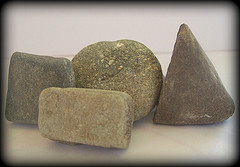Math 9 Honours Chapter 1:
PPT

•  1.1

•  1.2
•  1.3

•  1.4

•  1.5

Surface Area and Square Roots

In this section, students will learn to:

• Display an understanding of Rational and Irrational Numbers
Click for Homework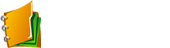Math 9 Honours Chapter 2:

PPT

•  2.1
•  2.2

•  2.3

•  2.4

•  2.5
•  2.6
•  2.7
•  Rev.
Powers and Exponent Laws

In this section, students will learn to:

• Compare rational numbers with different values
• Evaluate the square root of rational numbers that are perfect squares
• Approximate the Square root of rational numbers that are not perfect squares
• Solve problems involving rational numbers
Click for Homework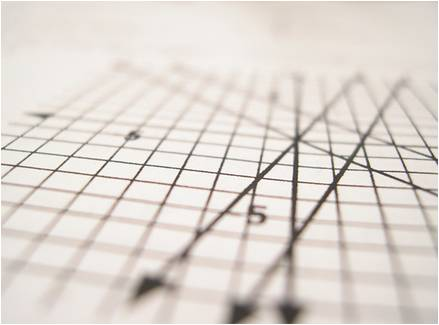Math 9 Honours Chapter 3:
PPT

•  3.1
•  3.2
•  3.3

•  3.4
•  3.5
•  3.6
•  3.7
•  Rev.

Linear Relations

In this chapter, students will learn:

to create a Table of Values for a linear function
to graph lines using slopes and y-intercepts
• to recognize equations for parallel and perpendicular lines
to algebraically find the intersection between two lines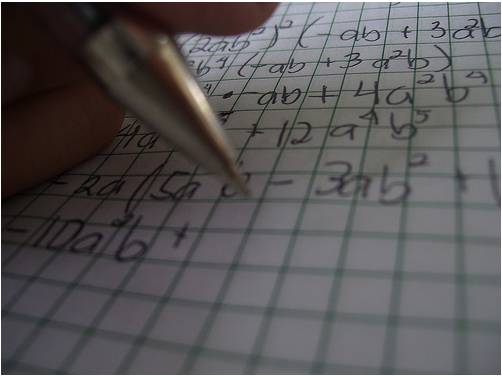Math 9 Honours Chapter 4:
PPT

•  4.1

•  4.2

•  4.3

•  4.4

•  4.5

•  4.6
•  4.7
•  4.8
•  Rev1
•  Rev.

Polynomials

This is a difficult and exciting chapter for most grade 9 honours students. Initially, students will learn basic computations with polynomial expressions like adding, subtracting, multiplying, and dividing polynomials. Later, we eventually explore complex methods of factoring and solving trinomials and polynomials with more than 3 terms. These methods include facctoring a difference of squares, difference of powers, completing the square, quadratic formula, and long division.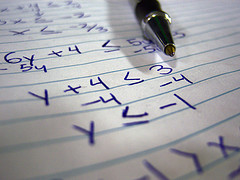Math 9 Honours Chapter 5:
Notes

•  5.1

•  5.2
•  5.3

•  5.4

•  5.5

•  Rev.

Linear Inequalities

In this chapter, students will learn:

• to graph a linear inequality on a number line
• to graph linear systems on a coordinate plane with two or more lines
to model and solve real life scenarios using systems of inequalities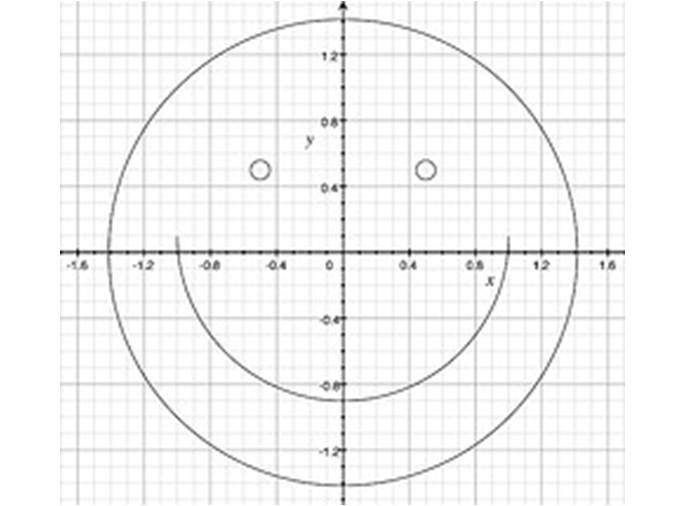Math 9 Honours Chapter 6:
PPT

•  6.1
•  6.2
•  6.3

•  6.4
•  6.4b
•  6.5
•  6.6
•  6.7
•  C6R
Similiarity and Trigonometry

In this chapter, students will learn:

• to understand the applications and purposes of trigonometry
• to draw connections between the ratios of the sides in a triangle with the degrees of its interior angles
to apply the cosine and sine law to solve for each side and angle of any triangle
Click for Homework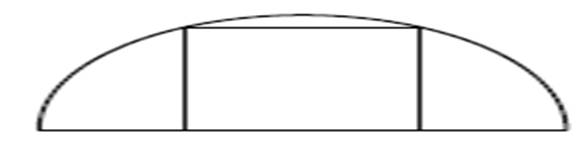Math 9 Honours Chapter 7:

PPT

•  7a
•  7.1
•  7.2

•  7.3
•  7.4
•  7.5

•  7.6
•  7.7

•  Rev.
Circle Geometry

In this chapter, students will learn:

• to about the properties of chords and angles in a circle
to prove a theorem by using properties of circle geometry
Click for HomeworkMath 9 Honours Chapter 8:

•  Section 8.1 Introduction to Non-Linear Functions
•  Section 8.2 Function Notations
•  Section 8.3 Domain and Range
•  Ch8 In Class Notes (ONE NOTE)

•  8.1

•  8.2
•  8.3

Functions

In this chapter, students will learn:

• to draw connections between the image of a
non-linear function and it's equation

• to simplify functions notations

to find the domain and range for a function